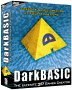# Physics - Dynamics

In the previous kinematics page the mathematics of moving objects was discussed. If the objects are representing physical objects, with properties like mass and inertia, then we probably want to calculate the kinematics from the dynamics

• Forward Dynamics - The movements are calculated from the forces, such as, force = mass * acceleration.
• Inverse Dynamics - Constraints are applied which specifies how objects interact, for example, they may be linked by a hinge joint or a ball joint, and from this the forces can be calculated (see joints).

What I would like to do in these pages about dynamics is to develop general equations for the motion of solid objects. So, given knowledge of the forces acting on the object and information about objects that it collides with, we will be able to predict its future motion.

From this I have suggested some structures for modeling this motion, and from this some computer programs.

Generalised equations for motion and collisions are quite complicated so, I propose to start by fully understanding linear motion, then add rotational motion, then add collisions.

## Forward Dynamics

For applications such as games and simulations of normal objects we can use Newtonian mechanics (as opposed to relativity or quantum mechanics)

Newton defines 3 laws (here defined in terms of particles):

1. If no forces act on a particle, the particle retains its linear momentum.
2. The rate of change of the linear momentum of a particle is equal to the sum of all forces acting on it.
3. When two particles exert forces upon each other, these forces are equal in magnitude and opposite in direction.

These laws can also be applied to rigid bodies by assuming that the forces are acting on the centre of mass of the object. Assuming that the mass is constant then the second law becomes:

force = mass * acceleration

For example, if the object is under the influence of gravity then: force due to gravity: force in Newtons= mass * 9.81

Euler extended these laws to include rotation. So there are equivalent laws for rotation such as:

torque = inertia * angular acceleration.

When working in three dimensions we can formulate these equations using vector and matrix notation, (see inertia).

When a solid object is moving in empty space with no external forces acting on it, then we can consider its linear velocity and its angular velocity independently:

• The linear velocity of the centre-of-mass will be constant.
• The angular velocity about the centre-of-mass will be constant, and if the object is not symmetrical, this rotation will always be about one of its Principal Moments of Inertia.

So here is a difference between linear and angular motion, with linear motion (provided there is no air resistance) it can move in all directions equally, regardless of the shape of the object. With rotation the direction of spin may be dependent on the orientation of the object (and visa versa).

There are other differences between linear motion and rotation, for instance, Newtonian laws can be applied in local coordinates when the local frame of reference has linear motion. However Newtonian laws don't work on a rotating frame of reference.

When we are dealing with rotation, some of these laws are not immediately initiative, there is no reason to give gyroscopes, for example, mystical significance, they are obeying simple laws (even if I don't claim to fully understand them yet!). However we can be fooled by our intuition into misunderstanding these rules. I am therefore collecting some simple examples here, table top physics, to help us get an initiative understanding. For example, too think about how an object rotates about its principle moment of inertia, see the egg example.

## Next Step and Further Reading

To see how an algorithm for implementing the dynamics of a solid object see here.

To see a proposal for a structure to hold this dynamics information see here.

Next: Linear motion

Where I can, I have put links to Amazon for books that are relevant to the subject, click on the appropriate country flag to get more details of the book or to buy it from them.Physics for Game Developers - Assumes a knowledge of vectors, Matrix and trigonometry (the book has a one page introduction to quatnions). The book introduces Newtons laws but it does assume a basic knowledge physics. It covers Kinematics, Force, Kinetics, Collision (detection), Projectiles, Aircraft, Ships, Hovercraft, Cars, Real-time, 2D rigid body, Collision Response, Rigid body rotation, 3D rigid body, multiple bodies in 3D and particles. (I cant find a general formula for collision response which combines linear and rotation, but there may be something in the code included?). If you don't have the prerequisite knowledge of Matrices etc. you may want to get the Mathematics for 3D Game programming book first.

Commercial Software Shop

Where I can, I have put links to Amazon for commercial software, not directly related to the software project, but related to the subject being discussed, click on the appropriate country flag to get more details of the software or to buy it from them.Dark Basic Professional Edition - It is better to get this professional editionThis is a version of basic designed for building games, for example to rotate a cube you might do the following: make object cube 1,100 for x=1 to 360 rotate object 1,x,x,0 next x

This site may have errors. Don't use for critical systems.